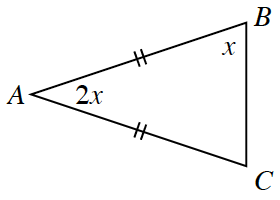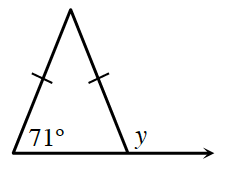### Home > INT2 > Chapter 2 > Lesson 2.2.1 > Problem2-56

2-56.

For each triangle below, solve for the variable and answer the questions.1. Solve for $x$.  What kind of triangle is $∆ABC$? Be specific.

What is true about the base angles of isosceles triangles?

$x = 45°$

2. Solve for $y$.  What kind of triangle is shown in the figure? Be specific.

Notice the supplementary angle with $y$. A supplementary angle adds up to $180^\circ$. Can you form an equation to solve for $y$?